# Free online courseCalculus 2 by Leonard

4.75

(8)

## 50h03m of online video course

Quality content and selected for your learning.

## Exercises to train your knowledge

Many of the courses available have exercises to help with learning.

This course is free today and always will be, from start to finish you will pay nothing.

## Calculus

You can use our courses of Calculus in a lot of ways, like in physics, mathematics, economics and many other and here for sure is the best place to learnTeacher

##### Leonard
###### 6 Courses / 1743 students

This Channel is dedicated to quality mathematics education. It is absolutely FREE so Enjoy! Videos are organized in playlists and are course specific.

## Course content preview

There are no comments yet for this course.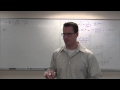Watch the 1st video of the course

## Course content2h22m

### Calculus 2 Lecture 6.1: The Natural Log Function

Calculus 2 Lecture 6.1: The Natural Log Function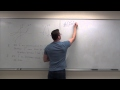0h44m

### Calculus 2 Lecture 6.2: Derivatives of Inverse Functions

Calculus 2 Lecture 6.2: Derivatives of Inverse Functions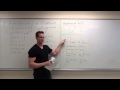1h30m

### Calculus 2 Lecture 6.3: Derivatives and Integrals of Exponential Functions

Calculus 2 Lecture 6.3: Derivatives and Integrals of Exponential Functions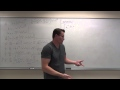1h15m

### Calculus 2 Lecture 6.4: Derivatives and Integrals of General Exponential Functions

Calculus 2 Lecture 6.4: Derivatives and Integrals of General Exponential Functions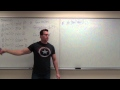1h52m

### Calculus 2 Lecture 6.5: Calculus of Inverse Trigonometric Functions

Calculus 2 Lecture 6.5: Calculus of Inverse Trigonometric Functions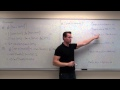1h16m

### Calculus 2 Lecture 6.6: A Discussion of Hyperbolic Functions

Calculus 2 Lecture 6.6: A Discussion of Hyperbolic Functions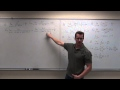1h40m

### Calculus 2 Lecture 6.7: Evaluating Limits of Indeterminate Forms

Calculus 2 Lecture 6.7: Evaluating Limits of Indeterminate Forms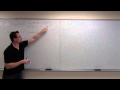1h54m

### Calculus 2 Lecture 7.1: Integration By Parts

Calculus 2 Lecture 7.1: Integration By Parts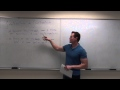2h21m

### Calculus 2 Lecture 7.2: Techniques For Trigonometric Integrals

Calculus 2 Lecture 7.2: Techniques For Trigonometric Integrals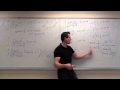2h09m

### Calculus 2 Lecture 7.3: Integrals By Trigonometric Substitution

Calculus 2 Lecture 7.3: Integrals By Trigonometric Substitution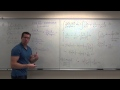2h55m

### Calculus 2 Lecture 7.4: Integration By Partial Fractions

Calculus 2 Lecture 7.4: Integration By Partial Fractions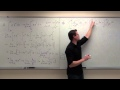2h48m

### Calculus 2 Lecture 7.6: Improper Integrals

Calculus 2 Lecture 7.6: Improper Integrals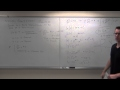2h49m

### Calculus 2 Lecture 8.1: Solving First Order Differential Equations By Separation of Variables

Calculus 2 Lecture 8.1: Solving First Order Differential Equations By Separation of Variables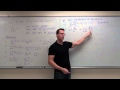2h27m

### Calculus 2 Lecture 9.1: Convergence and Divergence of Sequences

Calculus 2 Lecture 9.1: Convergence and Divergence of Sequences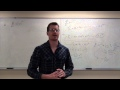2h01m

### Calculus 2 Lecture 9.2: Series, Geometric Series, Harmonic Series, and Divergence Test

Calculus 2 Lecture 9.2: Introduction to Series, Geometric Series, Harmonic Series, and the Divergence Test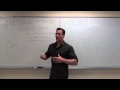1h11m

### Calculus 2 Lecture 9.3: Using the Integral Test for Convergence/Divergence of Series, P-Series

Calculus 2 Lecture 9.3: Using the Integral Test for Convergence/Divergence of Series, P-Series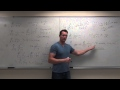1h34m

### Calculus 2 Lecture 9.4: The Comparison Test for Series and The Limit Comparison Test

Calculus 2 Lecture 9.4: The Comparison Test for Series and The Limit Comparison Test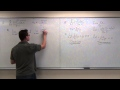1h19m

### Calculus 2 Lecture 9.5: Showing Convergence With the Alternating Series Test, Finding Error of Sums

Calculus 2 Lecture 9.5: Showing Convergence With the Alternating Series Test, Finding Error of Sums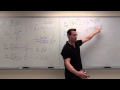1h46m

### Calculus 2 Lecture 9.6: Absolute Convergence, Ratio Test and Root Test For Series

Calculus 2 Lecture 9.6: Absolute Convergence, Ratio Test and Root Test For Series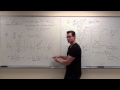2h29m

### Calculus 2 Lecture 9.7: Power Series, Calculus of Power Series, Ratio Test for Int. of Convergence

Calculus 2 Lecture 9.7: Power Series, Calculus of Power Series, Using Ratio Test to Find Interval of Convergence